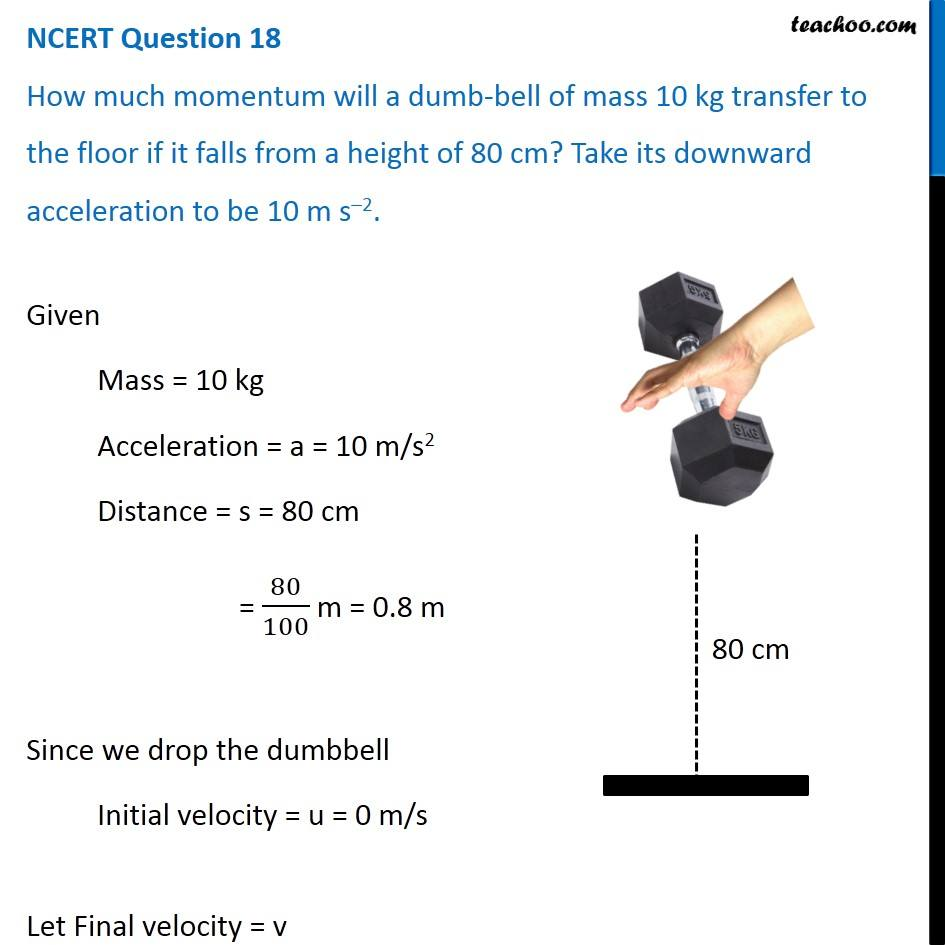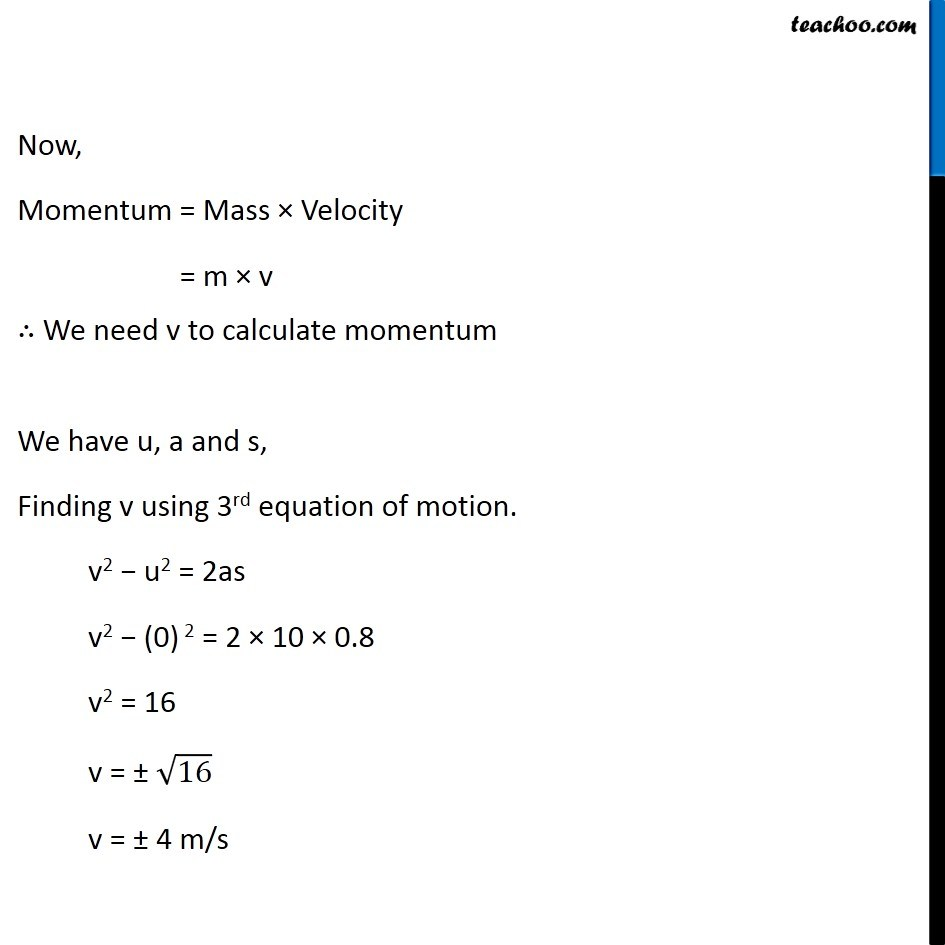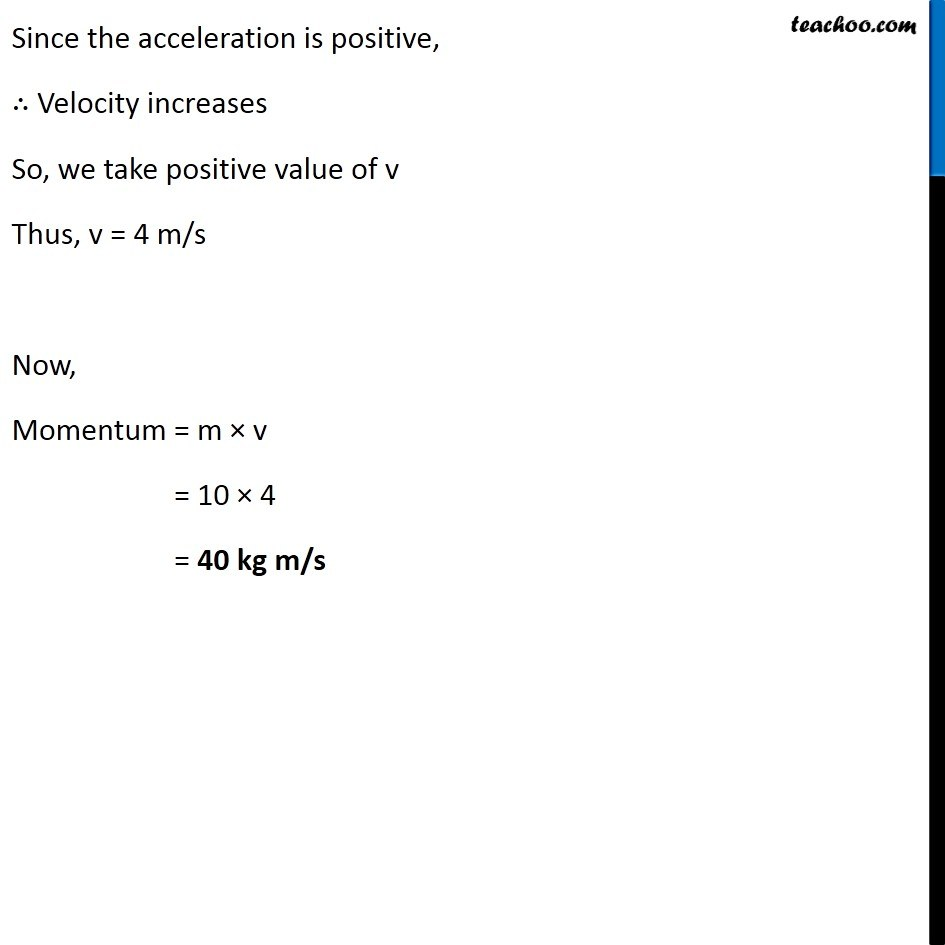NCERT Questions

Class 9
Chapter 9 Class 9 - Force and Laws Of MotionLearn in your speed, with individual attention - Teachoo Maths 1-on-1 Class

### Transcript

NCERT Question 18 How much momentum will a dumb-bell of mass 10 kg transfer to the floor if it falls from a height of 80 cm? Take its downward acceleration to be 10 m s–2. NCERT Question 18 How much momentum will a dumb-bell of mass 10 kg transfer to the floor if it falls from a height of 80 cm? Take its downward acceleration to be 10 m s–2. Now, Momentum = Mass × Velocity = m × v ∴ We need v to calculate momentum We have u, a and s, Finding v using 3rd equation of motion. v2 − u2 = 2as v2 − (0) 2 = 2 × 10 × 0.8 v2 = 16 v = ± √16 v = ± 4 m/s Since the acceleration is positive, ∴ Velocity increases So, we take positive value of v Thus, v = 4 m/s Now, Momentum = m × v = 10 × 4 = 40 kg m/s